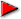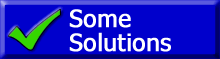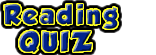ChaptersNext PageThis page contains a series of video tutorials about Kirchoff's rules for electrical electrical circuits. For this to make sense, you should have the basics skills listed below.

Necessary Background SkillsIdentify resistors in parallel or series (Actually this video discusses capacitors in series and parallel. But the rules are the same for identifying capacitors or resistors in parallel.)Reduce resistors in parallel to a single resistorReduce resistors in series to a single resistorIdentify what is the same for all resistors in series or parallelKirchoff's 1st Rule: the "Junction Rule"

http://youtu.be/XBYP69m4OF0

 Kirchoff's 2nd Rule: the "Loop Rule"

Video above is here: http://youtu.be/LmDLJJAlu_8

 Example 1 ...of the "Loop Rule"

Here is the video above: http://youtu.be/5oXJMgMVfOo

 Example 2 ...Using Both Rules

This example is shown in 3 videos. Below is part 1.

The video above is here: http://youtu.be/CW23eDhRYGQ

Below is part 2

The video is here: http://youtu.be/LAvwHgighHI

Below is part 3

The video is here: http://youtu.be/vddfsexfFOU

 Using the TI-83 or TI-84

This video will show how to use your TI-83, TI-83+, TI-84, or TI-84+ calculator to solve for multiple unknowns with an equal number of equations. This uses "Reduced Row Echelon Form," "rref."

This video is here: http://youtu.be/kXSipE2QePsThsi quiz assumes the defintion of a current as applied by physicists. Inother words energy always FLOWS from high potential energy to lower potential energy. This means that energy flows from the positive side of hte battery to the negative side. This is the same flow pattern that is described by the flow of positive charge.

by Tony Wayne ...(If you are a teacher, please feel free to use these resources in your teaching.)